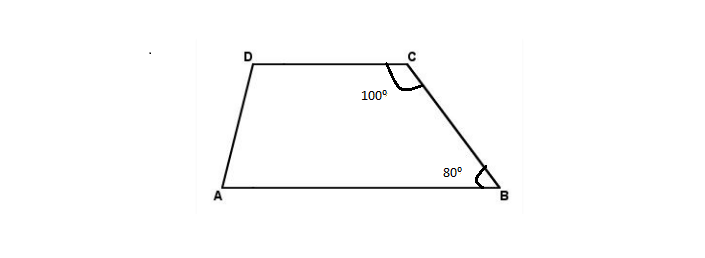# Explain How This Figure Is A Trapezium. Which Of Its Two Sides Are Parallel?Here,

\(= angle C + angle D \) \(=100^{circ} + 80^{circ} \) \(=180^{circ} \)

The sum of interior opposite angles is \(=180^{circ} \)

Therefore, BC and AD are parallel and ABCD is a Trapezium

Explore more such questions and answers at BYJU’S.